Featured

# Pie Chart In ASP.Net

Sometimes we need to show data in a chart like a Pie chart, such as to show quarterly data and on, so by considering the preceding requirement and to introduce the ASP.Net Pie Chart controls I have decided to write this article.
Let us learn about the ASP.Net chart type Pie chart that provides a powerful UI and design quality. We will learn about these chart type controls step-by-step. All the charts are in the System.Web.UI.DataVisualization.Charting namespace.
Chart data is represented using the following points:
1. X Axis: the horizontal line of the chart termed the X axis
2. Y Axis: the vertical line of the chart termed the Y axis

Now let us learn about the properties of the Pie chart. A Pie chart type has the following common properties:
• AlternetText: Sets the alternate text when the image is not available
• Annotation: Stores the chart annotations
• AntiAliasing: sets a value that determines whether anti-aliasing is used when text and graphics are drawn
• BackGradientStyle: sets the orientation for the background gradient for the Chart control. Also determines whether a gradient is used, the default is None
• Backcolor: sets the background color for a chart, the default color is White
• BackImage: sets the background image for the chart control.
• BackHatchStyle: sets the hatching style for the chart control, the default is None.
• Height: Sets the height for the chart control
• Width: Sets the width for the chart control
• Palette: Sets the style with the color for the chart control, the default style is Chocolate.
• PaletteCustomColors: Sets the custom color for the chart control.
• Series: Sets the series collection for the chart control
• Legends: Sets the series of legends to the chart
Now let us show the preceding explanation with a practical example by creating a simple web application.

#### Step 1: Create the table for the chart data

Now before creating the application, let us create a table named QuarterwiseSale in a database from where we show the records in the chart using the following script:

```CREATE TABLE [dbo].[QuarterwiseSale](
[id] [int] IDENTITY(1,1) NOT NULL,
[Quarter] [varchar](50) NULL,
[SalesValue] [money] NULL,
CONSTRAINT [PK_QuarterwiseSale] PRIMARY KEY CLUSTERED
(
[id] ASC
)WITH (PAD_INDEX = OFF, STATISTICS_NORECOMPUTE = OFF, IGNORE_DUP_KEY = OFF, ALLOW_ROW_LOCKS = ON, ALLOW_PAGE_LOCKS = ON) ON [PRIMARY]
) ON [PRIMARY]
```

The table has the following fields (shown in the following image):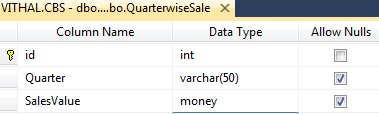Now insert some records using the following script:

```SET IDENTITY_INSERT [dbo].[QuarterwiseSale] ON

GO
INSERT [dbo].[QuarterwiseSale] ([id], [Quarter], [SalesValue]) VALUES (1, N'Q1', 100.0000)
GO
INSERT [dbo].[QuarterwiseSale] ([id], [Quarter], [SalesValue]) VALUES (2, N'Q2', 50.0000)
GO
INSERT [dbo].[QuarterwiseSale] ([id], [Quarter], [SalesValue]) VALUES (3, N'Q3', 150.0000)
GO
INSERT [dbo].[QuarterwiseSale] ([id], [Quarter], [SalesValue]) VALUES (4, N'Q4', 200.0000)
GO
SET IDENTITY_INSERT [dbo].[QuarterwiseSale] OFF
GO
```

Now the records will look as in the list in the following image: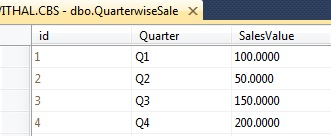Now create the Stored Procedure to fetch the records from database as in the following:

```Create Procedure [dbo].[GetSaleData]
(
@id int=null

)
as
begin
Select Quarter,SalesValue from QuarterwiseSale
End
```

I hope you have the same type of table and records as above.

#### Step: 2 Create Web Application

Now create the project using the following:
1. "Start" - "All Programs" - "Microsoft Visual Studio 2010".
2. "File" - "New Project" - "C#" - "Empty Project" (to avoid adding a master page).
3. Provide the project a name such as UsingPieChart or another as you wish and specify the location.
4. Then right-click on Solution Explorer and select "Add New Item" then select Default.aspx page.
5. Drag and Drop a Chart control from the ToolBox onto the Default.aspx page.
Now the Default.aspx source code will be as follows:

```<%@ Page Language="C#" AutoEventWireup="true" CodeFile="Default.aspx.cs" Inherits="_Default" %>

<%@ Register Assembly="System.Web.DataVisualization, Version=4.0.0.0, Culture=neutral, PublicKeyToken=31bf3856ad364e35"
Namespace="System.Web.UI.DataVisualization.Charting" TagPrefix="asp" %>
<!DOCTYPE html PUBLIC "-//W3C//DTD XHTML 1.0 Transitional//EN" "http://www.w3.org/TR/xhtml1/DTD/xhtml1-transitional.dtd">
<html xmlns="http://www.w3.org/1999/xhtml">
<body bgcolor="Navy">
<form id="form1" runat="server">
<h4 style="color: White;">
Article for C#Corner
</h4>
<asp:Chart ID="Chart1" runat="server" BackColor="0, 0, 64" BackGradientStyle="LeftRight"
BorderlineWidth="0" Height="360px" Palette="None" PaletteCustomColors="Maroon"
Width="380px" BorderlineColor="64, 0, 64">
<Titles>
</Titles>
<Legends>
<asp:Legend Alignment="Center" Docking="Bottom" IsTextAutoFit="False" Name="Default"
LegendStyle="Row" />
</Legends>
<Series>
<asp:Series Name="Default" />
</Series>
<ChartAreas>
<asp:ChartArea Name="ChartArea1" BorderWidth="0" />
</ChartAreas>
</asp:Chart>
</form>
</body>
</html>
```

Create a method to bind the chart control. Then open the default.aspx.cs page and create the following function named Bindchart to bind the Chart Control as in the following:

```private void Bindchart()
{
connection();
com = new SqlCommand("GetSaleData", con);
com.CommandType = CommandType.StoredProcedure;
DataSet ds = new DataSet();
da.Fill(ds);

DataTable ChartData = ds.Tables;

//storing total rows count to loop on each Record
string[] XPointMember = new string[ChartData.Rows.Count];
int[] YPointMember = new int[ChartData.Rows.Count];

for (int count = 0; count < ChartData.Rows.Count; count++)
{
//storing Values for X axis
XPointMember[count] = ChartData.Rows[count]["Quarter"].ToString();
//storing values for Y Axis
YPointMember[count] = Convert.ToInt32(ChartData.Rows[count]["SalesValue"]);

}
//binding chart control
Chart1.Series.Points.DataBindXY(XPointMember, YPointMember);

//Setting width of line
Chart1.Series.BorderWidth = 10;
//setting Chart type
Chart1.Series.ChartType = SeriesChartType.Pie;

foreach (Series charts in Chart1.Series)
{
foreach (DataPoint point in charts.Points)
{
switch (point.AxisLabel)
{
case "Q1": point.Color = Color.RoyalBlue; break;
case "Q2": point.Color = Color.SaddleBrown; break;
case "Q3": point.Color = Color.SpringGreen; break;
}
point.Label = string.Format("{0:0} - {1}", point.YValues, point.AxisLabel);

}
}

//Enabled 3D
//  Chart1.ChartAreas["ChartArea1"].Area3DStyle.Enable3D = true;
con.Close();

}
```

Note that we have written a small code snippet to give the different color for each point as in the following:

```//To give different color for each point
foreach (Series charts in Chart1.Series)
{
foreach (DataPoint point in charts.Points)
{
switch (point.AxisLabel)
{
case "Q1": point.Color = Color.RoyalBlue; break;
case "Q2": point.Color = Color.SaddleBrown; break;
case "Q3": point.Color = Color.SpringGreen; break;
}
point.Label = string.Format("{0:0} - {1}", point.YValues, point.AxisLabel);

}
}
```

Now, call the preceding function on page load as in the following:

```protected void Page_Load(object sender, EventArgs e)
{
if (!IsPostBack)
{
Bindchart();

}
}
```

The entire code of the default.aspx.cs page will look as follows:

```using System;
using System.Collections.Generic;
using System.Linq;
using System.Web;
using System.Web.UI;
using System.Web.UI.WebControls;
using System.Data.SqlClient;
using System.Configuration;
using System.Data;
using System.Web.UI.DataVisualization.Charting;
using System.Drawing;

public partial class _Default : System.Web.UI.Page
{
private SqlConnection con;
private SqlCommand com;
private string constr, query;
private void connection()
{
constr = ConfigurationManager.ConnectionStrings["getconn"].ToString();
con = new SqlConnection(constr);
con.Open();

}
protected void Page_Load(object sender, EventArgs e)
{
if (!IsPostBack)
{
Bindchart();

}
}

private void Bindchart()
{
connection();
com = new SqlCommand("GetSaleData", con);
com.CommandType = CommandType.StoredProcedure;
DataSet ds = new DataSet();
da.Fill(ds);

DataTable ChartData = ds.Tables;

//storing total rows count to loop on each Record
string[] XPointMember = new string[ChartData.Rows.Count];
int[] YPointMember = new int[ChartData.Rows.Count];

for (int count = 0; count < ChartData.Rows.Count; count++)
{
//storing Values for X axis
XPointMember[count] = ChartData.Rows[count]["Quarter"].ToString();
//storing values for Y Axis
YPointMember[count] = Convert.ToInt32(ChartData.Rows[count]["SalesValue"]);

}
//binding chart control
Chart1.Series.Points.DataBindXY(XPointMember, YPointMember);

//Setting width of line
Chart1.Series.BorderWidth = 10;
//setting Chart type
Chart1.Series.ChartType = SeriesChartType.Pie;

foreach (Series charts in Chart1.Series)
{
foreach (DataPoint point in charts.Points)
{
switch (point.AxisLabel)
{
case "Q1": point.Color = Color.RoyalBlue; break;
case "Q2": point.Color = Color.SaddleBrown; break;
case "Q3": point.Color = Color.SpringGreen; break;
}
point.Label = string.Format("{0:0} - {1}", point.YValues, point.AxisLabel);

}
}

//Enabled 3D
//  Chart1.ChartAreas["ChartArea1"].Area3DStyle.Enable3D = true;
con.Close();

}
}
```

We now have the entire logic to bind the chart from the database, let us run the application. The chart will look as follows: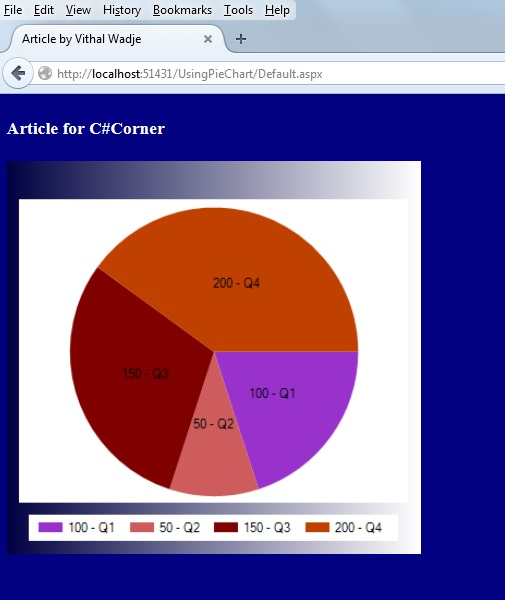Now let us change the Point color as:

```foreach (Series charts in Chart1.Series)
{
foreach (DataPoint point in charts.Points)
{
switch (point.AxisLabel)
{
case "Q1": point.Color = Color.YellowGreen; break;
case "Q2": point.Color = Color.Yellow; break;
case "Q3": point.Color = Color.SpringGreen; break;
}
point.Label = string.Format("{0:0} - {1}", point.YValues, point.AxisLabel);

}
}
```

Now the chart will look as follows: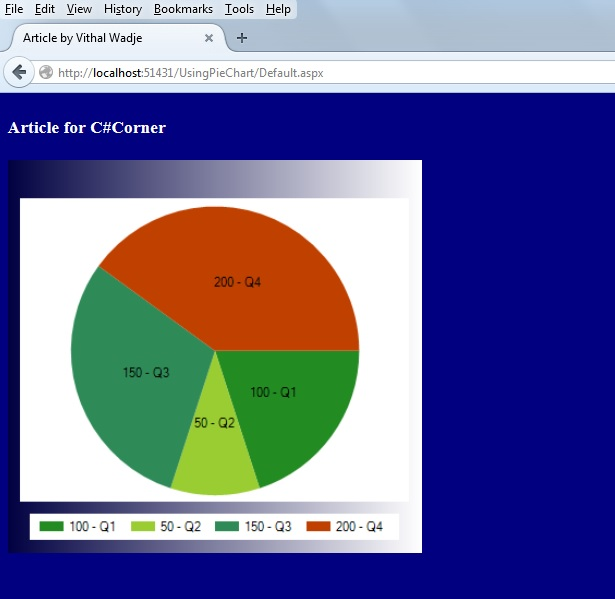In the preceding chart we saw how the data is properly arranged with the user interactive graphics, now let us set the 3D style enabled as in the following:

```Chart1.ChartAreas["ChartArea1"].Area3DStyle.Enable3D = true;
```

Now the chart will look as follows: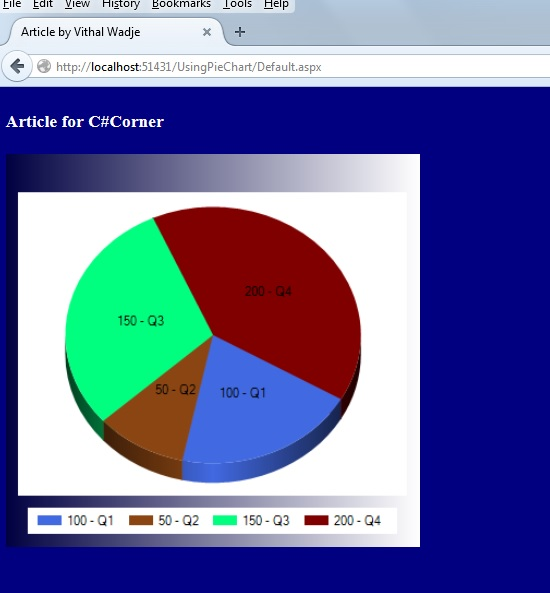Now change the Border width as:

```//Setting width of line
Chart1.Series.BorderWidth = 4;
```

Now the chart will look as follows: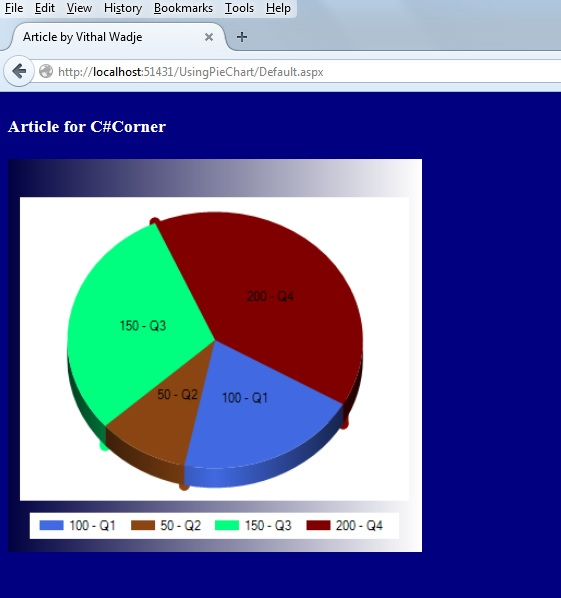Now from all the preceding explanations we saw how to create and use a Pie type chart.

Notes
• Change the connection string in the web.config file to specify your server location.